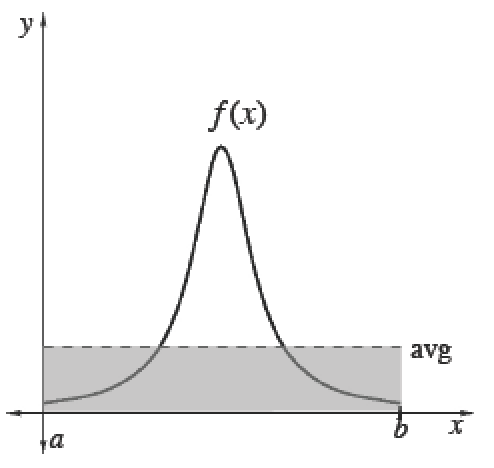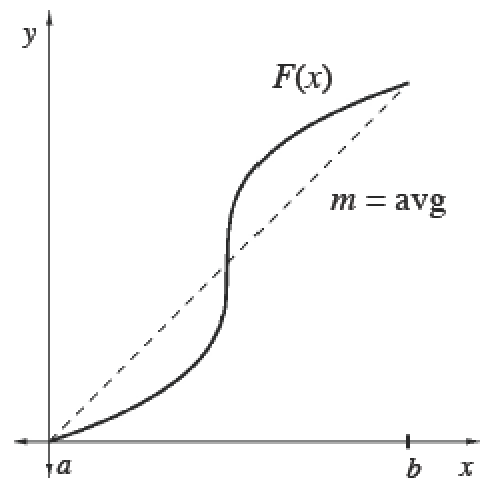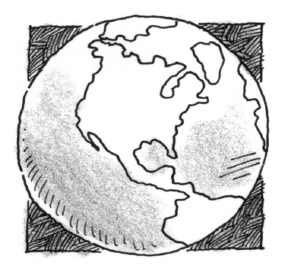### Home > APCALC > Chapter 7 > Lesson 7.1.3 > Problem7-33

7-33.

In order to plan for the future water supply, the average population of the world is needed. If the world population in 2015 was about $7.3$ billion people, determine the average population of the Earth during the next $30$ years. Assume the population, in billions, $t$ years from 2015 is projected to be:

$P(t)=7.3e^{0.014t}$

You are asked to determine the average value of $P(t)$, given the $P(t)$ function itself. Which variation of Mean Value will you use?

##### Average (Mean) Values
 To calculate the mean (average) value of a finite set of items, add up the values of items and divide by the number of items.Integrals help us add over a continuous interval. Therefore, for any continuous function $f$ :$\frac{\int_a^bf(x)dx}{b-a}=$ mean value of $f$ over $[a, b]$Since $\int _ { a } ^ { b } f ( x ) d x$, we can also calculate the average value of any function $f$ using its antiderivative $F$. Its average slope gives the average rate of change of $F$, which is the same as the average value of $f.$$\frac{\int_a^bf(x)dx}{b-a}=\frac { F ( b ) - F ( a ) } { b - a }=$  mean rate of change of $F ′$ over $[a, b]$